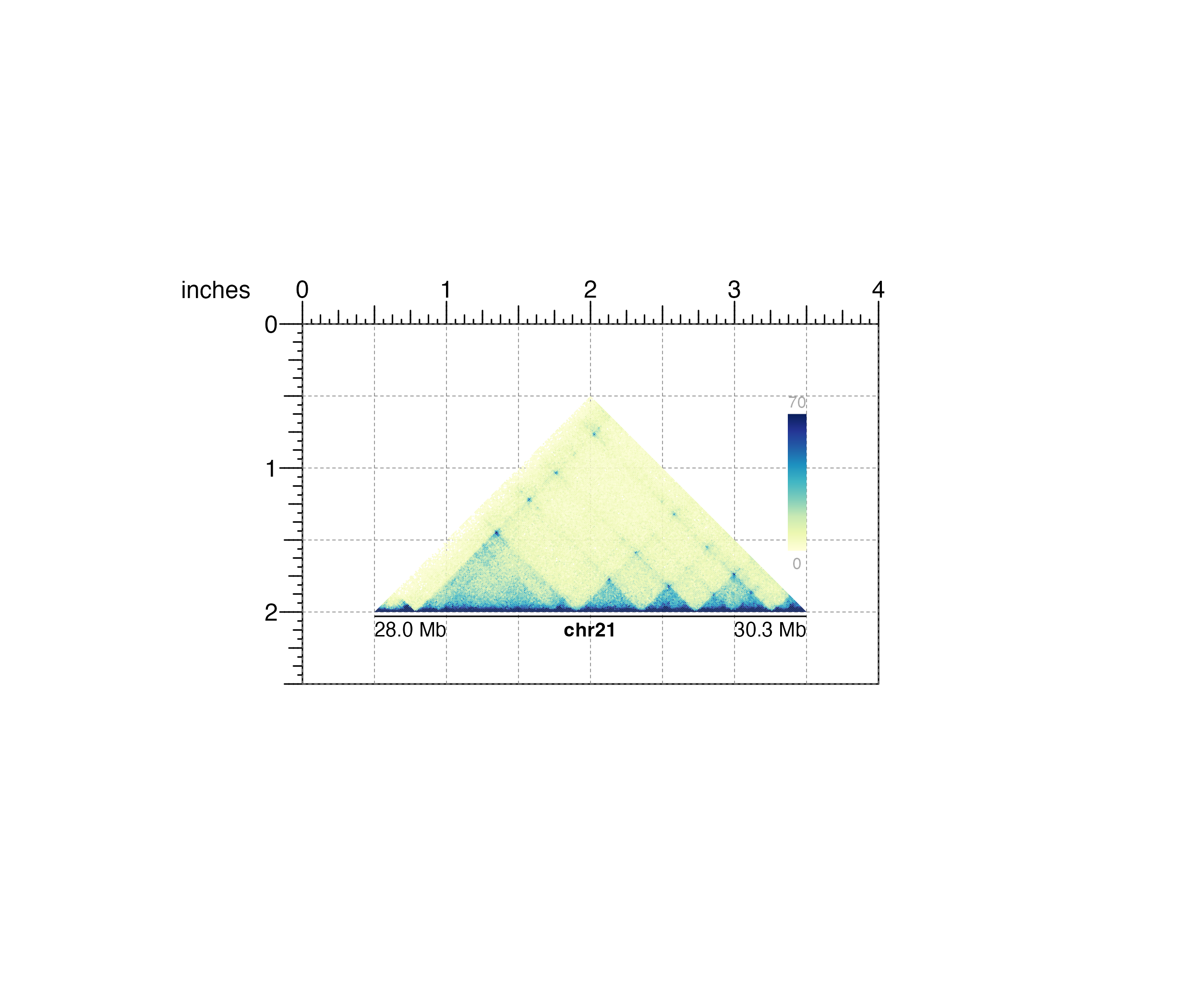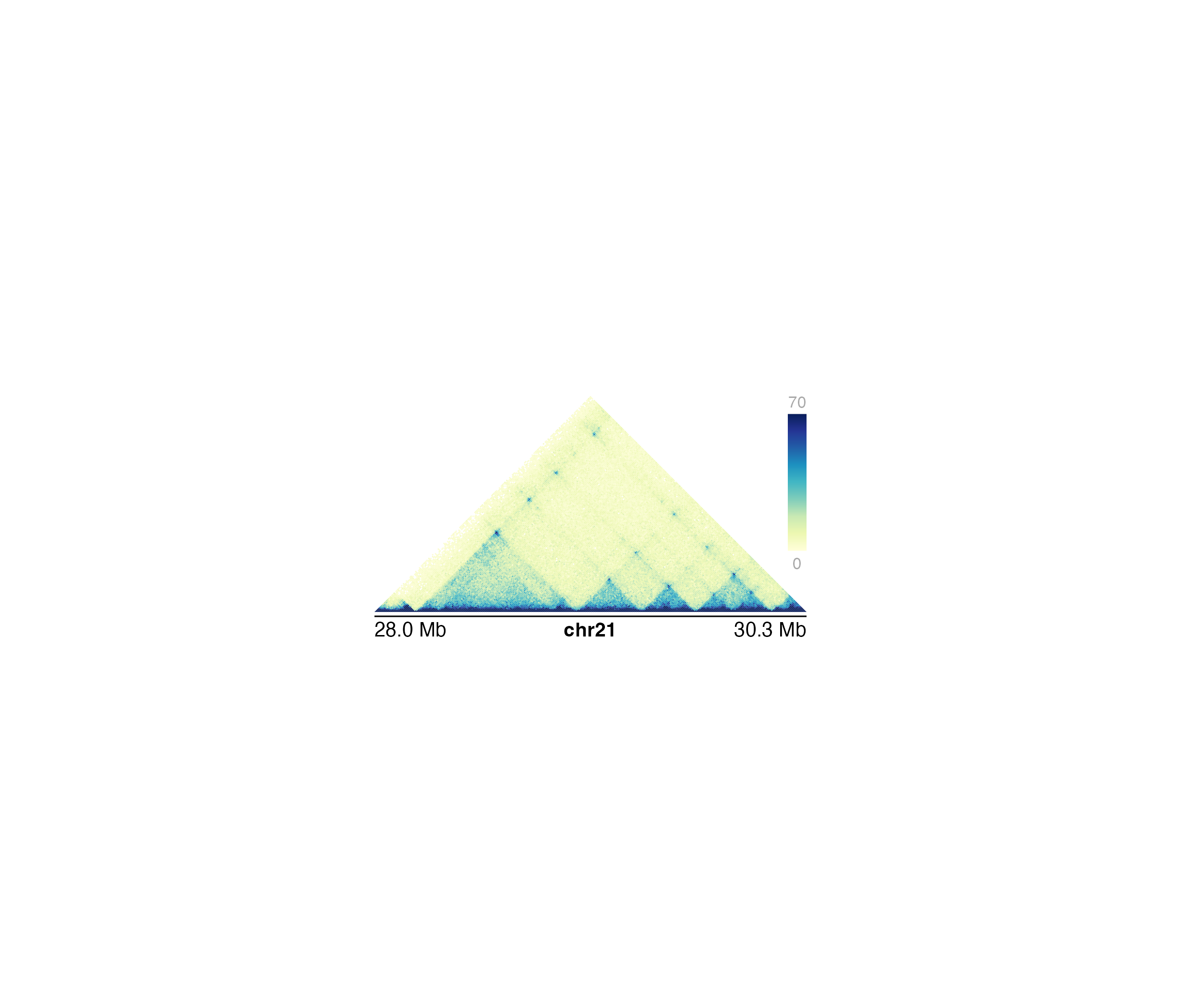Plot a Hi-C interaction matrix in a triangular format

plotHicTriangle(
data,
resolution = "auto",
zrange = NULL,
norm = "KR",
matrix = "observed",
chrom,
chromstart = NULL,
chromend = NULL,
assembly = "hg38",
palette = colorRampPalette(brewer.pal(n = 9, "YlGnBu")),
colorTrans = "linear",
flip = FALSE,
bg = NA,
x = NULL,
y = NULL,
width = NULL,
height = NULL,
just = c("left", "top"),
default.units = "inches",
draw = TRUE,
params = NULL,
quiet = FALSE
)

## Arguments

data

Path to .hic file as a string or a 3-column dataframe of interaction counts in sparse upper triangular format.

resolution

A numeric specifying the width in basepairs of each pixel. For hic files, "auto" will attempt to choose a resolution based on the size of the region. For dataframes, "auto" will attempt to detect the resolution the dataframe contains.

zrange

A numeric vector of length 2 specifying the range of interaction scores to plot, where extreme values will be set to the max or min.

norm

Character value specifying hic data normalization method, if giving .hic file. This value must be found in the .hic file. Default value is norm = "KR".

matrix

Character value indicating the type of matrix to output. Default value is matrix = "observed". Options are:

• "observed": Observed counts.

• "oe": Observed/expected counts.

• "log2oe": Log2 transformed observed/expected counts.

chrom

Chromosome of region to be plotted, as a string.

chromstart

Integer start position on chromosome to be plotted.

chromend

Integer end position on chromosome to be plotted.

assembly

Default genome assembly as a string or a assembly object. Default value is assembly = "hg38".

palette

A function describing the color palette to use for representing scale of interaction scores. Default value is palette = colorRampPalette(brewer.pal(n = 9, "YlGnBu")).

colorTrans

A string specifying how to scale Hi-C colors. Options are "linear", "log", "log2", or "log10". Default value is colorTrans = "linear".

flip

A logical indicating whether to flip the orientation of the Hi-C matrix over the x-axis. Default value is flip = FALSE.

bg

Character value indicating background color. Default value is bg = NA.

x

A numeric or unit object specifying triangle Hi-C plot x-location.

y

A numeric, unit object, or character containing a "b" combined with a numeric value specifying triangle Hi-C plot y-location. The character value will place the triangle Hi-C plot y relative to the bottom of the most recently plotted plot according to the units of the plotgardener page.

width

A numeric or unit object specifying the bottom width of the Hi-C plot triangle.

height

A numeric or unit object specifying the height of the Hi-C plot triangle.

just

Justification of triangle Hi-C plot relative to its (x, y) location. If there are two values, the first value specifies horizontal justification and the second value specifies vertical justification. Possible string values are: "left", "right", "centre", "center", "bottom", and "top". Default value is just = c("left", "top").

default.units

A string indicating the default units to use if x, y, width, or height are only given as numerics. Default value is default.units = "inches".

draw

A logical value indicating whether graphics output should be produced. Default value is draw = TRUE.

params

An optional pgParams object containing relevant function parameters.

quiet

A logical indicating whether or not to print messages.

## Value

Returns a hicTriangle object containing relevant genomic region, Hi-C data, placement, and grob information.

## Details

A triangle Hi-C plot can be placed on a plotgardener coordinate page by providing plot placement parameters:

plotHicTriangle(data, chrom,
chromstart = NULL, chromend = NULL,
x, y, width, height, just = c("left", "top"),
default.units = "inches")

This function can also be used to quickly plot an unannotated triangle Hi-C plot by ignoring plot placement parameters:

plotHicTriangle(data, chrom,
chromstart = NULL, chromend = NULL)

If height is $$<$$ $$0.5 * width$$, the top of the triangle will be cropped to the given height.

## Examples

## Load Hi-C data
library(plotgardenerData)
data("IMR90_HiC_10kb")

## Create a page
pageCreate(width = 4, height = 2.5, default.units = "inches")

## Plot and place triangle Hi-C plot
hicPlot <- plotHicTriangle(
data = IMR90_HiC_10kb, resolution = 10000,
zrange = c(0, 70),
chrom = "chr21",
chromstart = 28000000, chromend = 30300000,
assembly = "hg19", bg = "black",
x = 2, y = 0.5, width = 3, height = 1.5,
just = "top", default.units = "inches"
)
#> Read in dataframe.  Assuming 'chrom' in column1 and 'altchrom' in column2. 10000 BP resolution detected.
#> hicTriangle[hicTriangle1]

## Annotate x-axis genome label
annoGenomeLabel(
plot = hicPlot, scale = "Mb", x = 0.5, y = 2.03,
just = c("left", "top")
)
#> genomeLabel[genomeLabel1]

## Annotate heatmap legend
annoHeatmapLegend(
plot = hicPlot, x = 3.5, y = 0.5,
width = 0.13, height = 1.2,
just = c("right", "top")
)
#> heatmapLegend[heatmapLegend1]## Hide page guides
pageGuideHide()